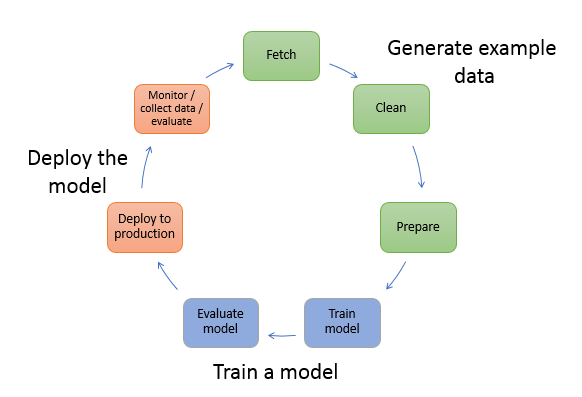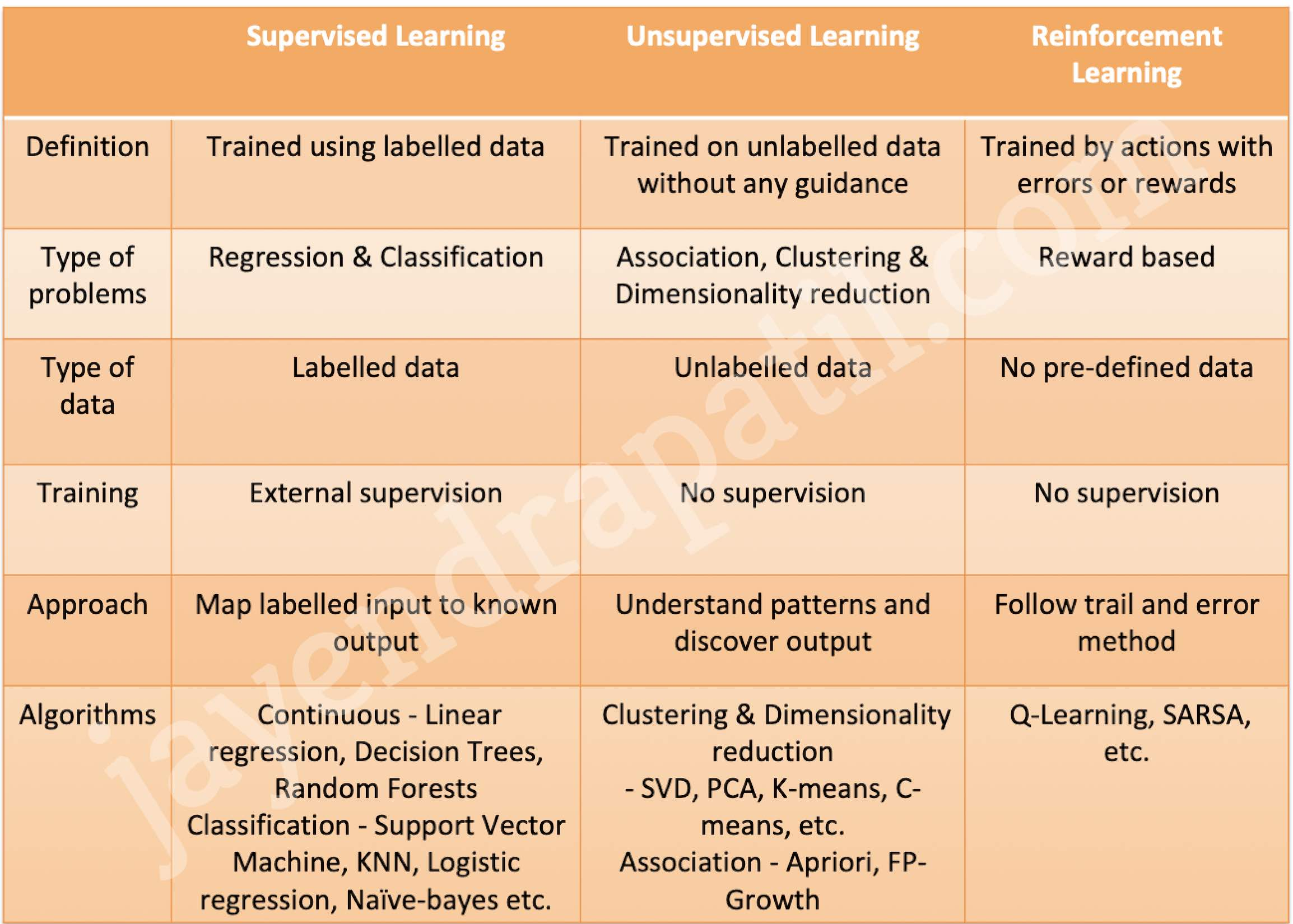# AWS Certification – Machine Learning Concepts – Cheat Sheet# Machine Learning Concepts

This post covers some of the basic Machine Learning concepts mostly relevant for the AWS Machine Learning certification exam.

## Machine Learning Lifecycle### Data Processing and Exploratory Analysis

• To train a model, you need data.
• Type of data that depends on the business problem that you want the model to solve (the inferences that you want the model to generate).
• Process data includes data collection, data cleaning, data split, data exploring, preprocessing, transformation, formatting etc.

#### Feature Selection and Engineering

• helps improve model accuracy and speed up training
• remove irrelevant data inputs using domain knowledge for e.g. name
• remove features which has same values, very low correlation, very little variance or lot of missing values
• handle missing data using mean values or imputation
• combine features which are related for e.g. height and age to height/age
• convert or transform features to useful representation for e.g. date to day or hour
• standardize data ranges across features

#### Missing Data

• do nothing
• remove the feature with lot of missing data points
• remove samples with missing data, if the feature needs to be used
• Impute using mean/median value
• no impact and the dataset is not skewed
• works with numerical values only. Do not use for categorical features.
• doesn’t factor correlations between features
• Impute using (Most Frequent) or (Zero/Constant) Values
• works with categorical features
• doesn’t factor correlations between features
• can introduce bias
• Impute using k-NN, Multivariate Imputation by Chained Equation (MICE), Deep Learning
• more accurate than the mean, median or most frequent
• Computationally expensive

#### Unbalanced Data

• Source more real data
• Oversampling instances of the minority class or undersampling instances of the majority class
• Create or synthesize data using techniques like SMOTE (Synthetic Minority Oversampling TEchnique)

#### Label Encoding and One-hot Encoding

• Models cannot multiply strings by the learned weights, encoding helps convert strings to numeric values.
• Label encoding
• Use Label encoding to provide lookup or map string data values to a numerical values
• However, the values are random and would impact the model
• One-hot encoding
• Use One-hot encoding for Categorical features that have a discrete set of possible values.
• One-hot encoding provide binary representation by converting data values into features without impacting the relationships
• a binary vector is created for each categorical feature in the model that represents values as follows:
• For values that apply to the example, set corresponding vector elements to 1.
• Set all other elements to 0.
• Multi-hot encoding is when multiple values are 1

#### Cleaning Data

• Scaling or Normalization means converting floating-point feature values from their natural range (for example, 100 to 900) into a standard range (for example, 0 to 1 or -1 to +1)

### Train a model

• Model training includes both training and evaluating the model,
• To train a model, algorithm is needed.
• Data can be split into training data, validation data and test data
• Algorithm sees and is directly influenced by the training data
• Algorithm uses but is indirectly influenced by the validation data
• Algorithm does not see the testing data during training
• Training can be performed using normal parameters or features and hyperparameters

## Supervised, Unsupervised and Reinforcement Learning#### Splitting and Randomization

• Always randomize the data before splitting

#### Hyperparameters

• influence how the training occurs
• Common hyperparameters are learning rate, epoch, batch size
• Learning rate – size of the step taken during gradient descent optimization
• Batch size
• number of samples used to train at any one time
• can be all (batch), one (stochastic) or some (mini batch)
• calculable from infrastructure
• Epochs
• number of times the algorithm processes the entire training data
• each epoch or run can see the model get closer to the desired state
• depends on algorithm used

### Evaluating the model

After training the model, evaluate it to determine whether the accuracy of the inferences is acceptable.

#### ML Model Insights

• For binary classification models use accuracy metric called Area Under the (Receiver Operating Characteristic) Curve (AUC). AUC measures the ability of the model to predict a higher score for positive examples as compared to negative examples.
• For regression tasks, use the industry standard root mean square error (RMSE) metric. It is a distance measure between the predicted numeric target and the actual numeric answer (ground truth). The smaller the value of the RMSE, the better is the predictive accuracy of the model.

#### Cross-Validation

• is a technique for evaluating ML models by training several ML models on subsets of the available input data and evaluating them on the complementary subset of the data.
• Use cross-validation to detect overfitting, ie, failing to generalize a pattern.
• there is no separate validation data, involves splitting the training data into chunks of validation data and use it for validation#### Optimization

• Gradient Descent is used to optimize many different types of machine learning algorithms
• Step size sets Learning rate
• If the learning rate is too large, the minimum slope might be missed and the graph would oscillate
• If the learning rate is too small, it requires too many steps which would take the process longer and is less efficient

#### Overfitting

• Simplify the model, by reducing number of layers
• Early Stopping – form of regularization while training a model with an iterative method, such as gradient descent
• Data Augmentation
• Regularization – technique to reduce the complexity of the model
• Dropout is a regularization technique that prevents overfitting

## Classification Model Evaluation

### Confusion Matrix

• Confusion matrix represents the percentage of times each label was predicted in the training set during evaluation
• An NxN table that summarizes how successful a classification model’s predictions were; that is, the correlation between the label and the model’s classification.
• One axis of a confusion matrix is the label that the model predicted, and the other axis is the actual label.
• N represents the number of classes. In a binary classification problem, N=2
• For example, here is a sample confusion matrix for a binary classification problem:
Tumor (predicted) Non-Tumor (predicted)
Tumor (actual) 18 (True Positives) 1 (False Negatives)
Non-Tumor (actual) 6 (False Positives) 452 (True Negatives)
• Confusion matrix shows that of the 19 samples that actually had tumors, the model correctly classified 18 as having tumors (18 true positives), and incorrectly classified 1 as not having a tumor (1 false negative).
• Similarly, of 458 samples that actually did not have tumors, 452 were correctly classified (452 true negatives) and 6 were incorrectly classified (6 false positives).
• Confusion matrix for a multi-class classification problem can help you determine mistake patterns. For example, a confusion matrix could reveal that a model trained to recognize handwritten digits tends to mistakenly predict 9 instead of 4, or 1 instead of 7.

### Accuracy, Precision, Recall (Sensitivity) and Specificity

#### Accuracy

• A metric for classification models, that identifies fraction of predictions that a classification model got right.
• In Binary classification, calculated as (True Positives+True Negatives)/Total Number Of Examples
• In Multi-class classification, calculated as Correct Predictions/Total Number Of Examples

#### Precision

• A metric for classification models. that identifies the frequency with which a model was correct when predicting the positive class.
• Calculated as True Positives/(True Positives + False Positives)

#### Recall – Sensitivity – True Positive Rate (TPR)

• A metric for classification models that answers the following question: Out of all the possible positive labels, how many did the model correctly identify i.e. Number of correct positives out of actual positive results
• Calculated as True Positives/(True Positives + False Negatives)
• Important when – False Positives are acceptable as long as ALL positives are found for e.g. it is fine to predict Non-Tumor as Tumor as long as All the Tumors are correctly predicted

#### Specificity – True Negative Rate (TNR)

• Number of correct negatives out of actual negative results
• Calculated as True Negatives/(True Negatives + False Positives)
• Important when – False Positives are unacceptable; it’s better to have false negatives for e.g. it is not fine to predict Non-Tumor as Tumor;

### ROC and AUC

#### ROC (Receiver Operating Characteristic) Curve

• An ROC curve (receiver operating characteristic curve) is curve of true positive rate vs. false positive rate at different classification thresholds.
• An ROC curve is a graph showing the performance of a classification model at all classification thresholds.
• An ROC curve plots True Positive Rate (TPR) vs. False Positive Rate (FPR) at different classification thresholds. Lowering the classification threshold classifies more items as positive, thus increasing both False Positives and True Positives.#### AUC (Area under the ROC curve)

• AUC stands for “Area under the ROC Curve.”
• AUC measures the entire two-dimensional area underneath the entire ROC curve (think integral calculus) from (0,0) to (1,1).
• AUC provides an aggregate measure of performance across all possible classification thresholds.
• One way of interpreting AUC is as the probability that the model ranks a random positive example more highly than a random negative example.### F1 Score

• F1 score (also F-score or F-measure) is a measure of a test’s accuracy.
• It considers both the precision p and the recall r of the test to compute the score: p is the number of correct positive results divided by the number of all positive results returned by the classifier, and r is the number of correct positive results divided by the number of all relevant samples (all samples that should have been identified as positive). we.

## Deploy the model

• Re-engineer a model before integrate it with the application and deploy it.
• Can be deployed as a Batch or as a Service
Posted in AWS

## One thought on “AWS Certification – Machine Learning Concepts – Cheat Sheet”

1.Dataexpert010 says:

Your amazing insightful information entails much to me and especially to my peers. Thanks a ton; from all of us.

This site uses Akismet to reduce spam. Learn how your comment data is processed.